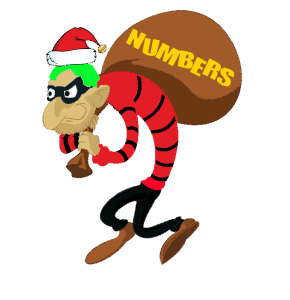# Mr. R.’s World of Math

## NUMBER THIEF MULTIPLICATION STORIESMultiply by 2 (doubles) Multiply By 3! Multiply By 4! Multiply By 5! Multiply By 6! Multiply By 7! Multiply By 8! (Halloween) Multiply By 9! Multiply By 10! Multiply By 11! Multiply By 12!